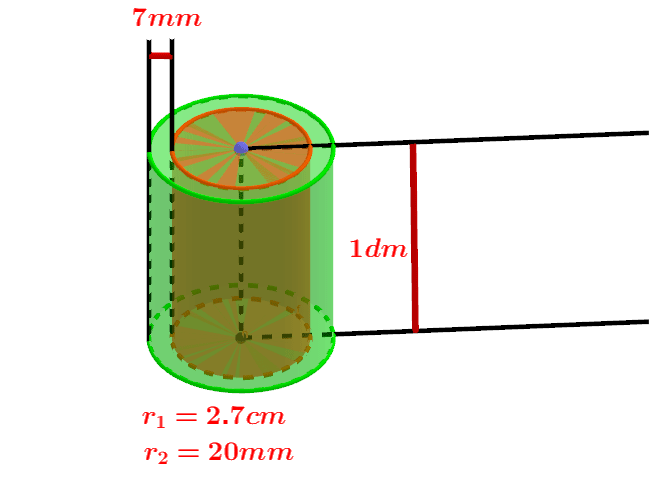Math Calculators, Lessons and Formulas

It is time to solve your math problem

mathportal.org
• Geometry
• Cylinder

# Cylinder

ans:
syntax error
C
DEL
ANS
±
(
)
÷
×
7
8
9
4
5
6
+
1
2
3
=
0
.
auto next question
calculator
•  Question 1: 1 pts Find the surface area of the cylinder shown on the picture.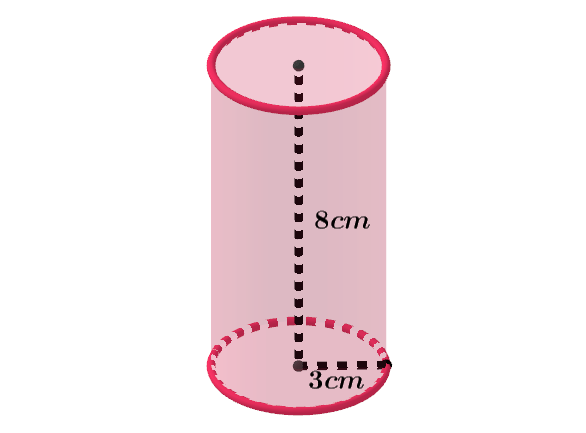$66\pi cm^{2}$ $72\pi cm^{2}$ $81\pi cm^{2}$
•  Question 2: 1 pts Find the surface area of the axial section of the cylinder shown on the picture.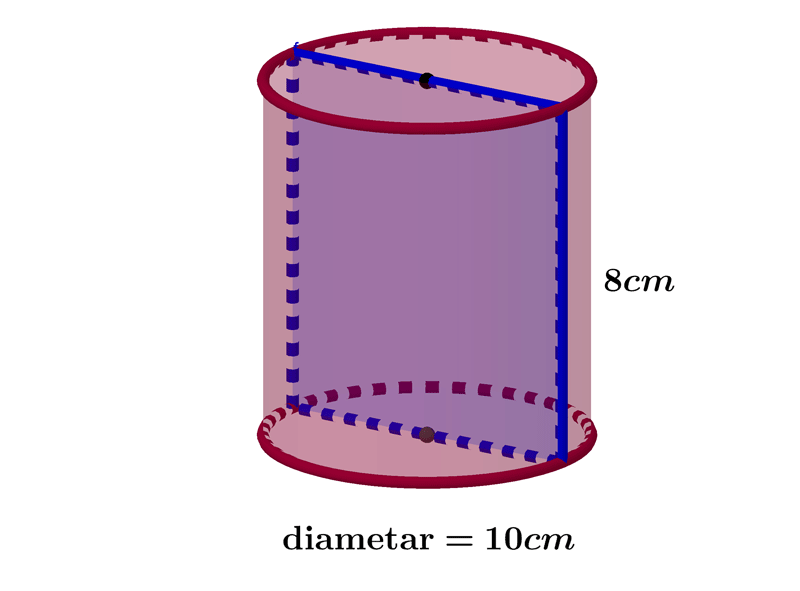$80cm\pi ^{2}$ $40cm\pi ^{2}$ $60cm^{2}$ $80cm^{2}$
•  Question 3: 1 pts The area of the base of the cylinder is $20$ square centimeters, and the lateral surface area is $100$ square centimeters. Find the total surface area of that cylinder.
 $130cm^{2}$ $140cm^{2}$ $120cm^{2}$ $110cm^{2}$
•  Question 4: 1 pts The area of the base of the cylinder is $50 cm^{2}$ and its height is $10cm.$ Find the volume of that cylinder.
 $250cm^{3}$ $\dfrac{500}{3}cm^{3}$ $\dfrac{250}{3}cm^{3}$ $500cm^{3}$
•  Question 5: 2 pts Find the volume of the cylinder shown on the picture.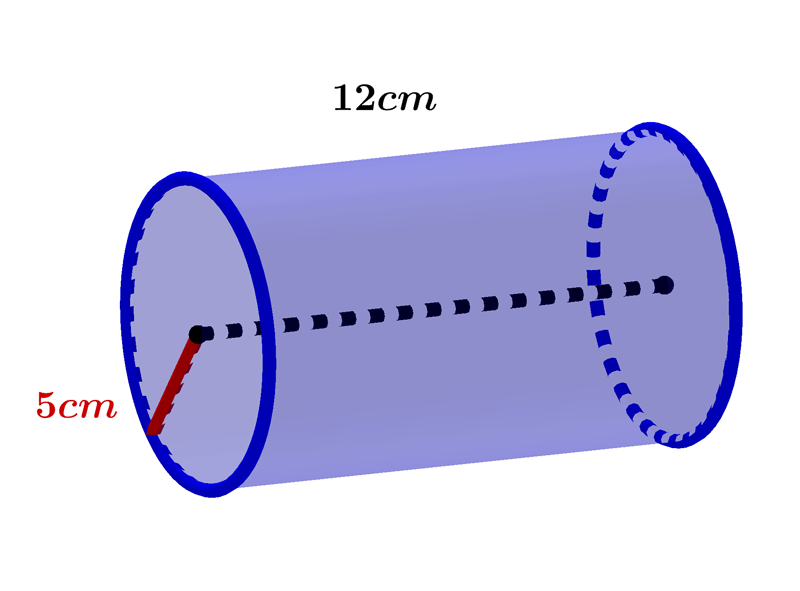Volume $=$
•  Question 6: 2 pts Find the volume of the cylinder If a height of a cylinder becomes $\dfrac{1}{4}$ of the original height and the radius is doubled, then which of the following will be true?
 Volume of the cylinder will be doubled. Volume of the cylinder will remain unchanged. Volume of the cylinder will halved. Volume of the cylinder will be $\dfrac{1}{4}$ of the original volume.
•  Question 7: 2 pts Find the volume of the cylinder shown on the picture.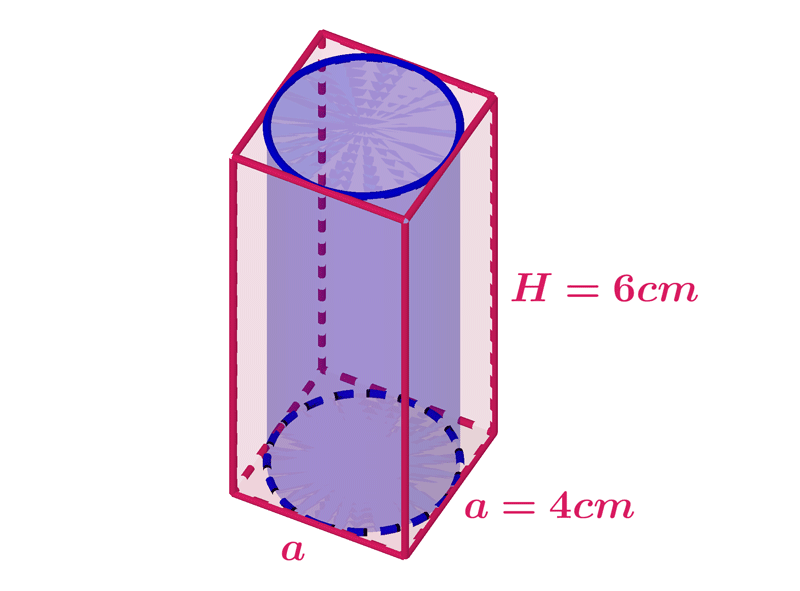$42\pi cm^{3}$ $36\pi cm^{2}$ $24\pi cm^{3}$
•  Question 8: 2 pts The surface area of the cylinder shown on the picture is $$A=\left(\left(7.6 \right)^{2}+7.6\cdot \pi \cdot 12.2\right)cm^{2}$$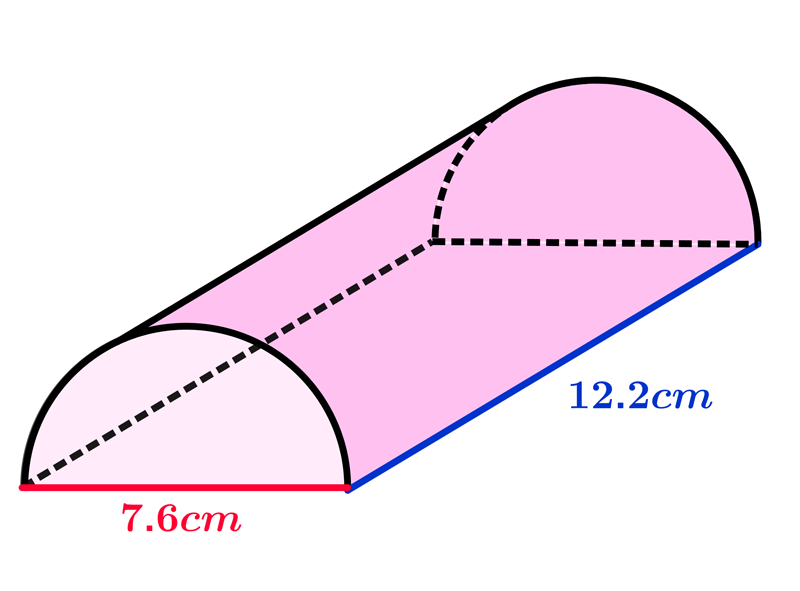•  Question 9: 3 pts Find the volume of the cylinder shown on the picture.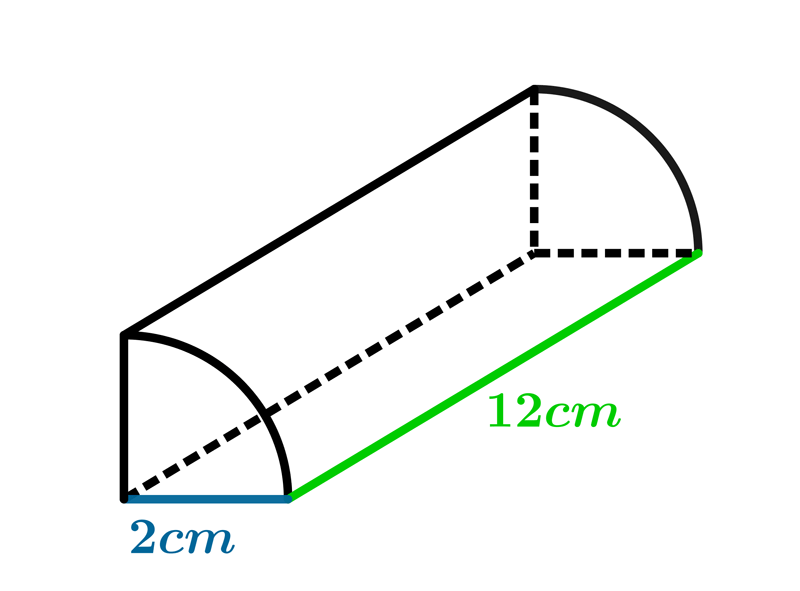Volume $=$
•  Question 10: 3 pts Mary is using a battery for her science project. The radius of the battery is 16 mm and it is 50mm tall. What is the volume of the battery?
 $7860\pi \, mm^{3}$ $10360\pi \, mm^{3}$ $12800\pi\, mm^{3}$ $12240\pi \,mm^{3}$
•  Question 11: 3 pts The ratio of radii of two cylinders is $1:3$, and heights are in ratio $1:4.$ Find the ratio of their volumes?
 $V_{1}:V_{2}=1:27$ $V_{1}:V_{2}=1:36$ $V_{1}:V_{2}=1:6$ $V_{1}:V_{2}=1:21$
•  Question 12: 3 pts Find the surface area of the cylinder shown on the picture.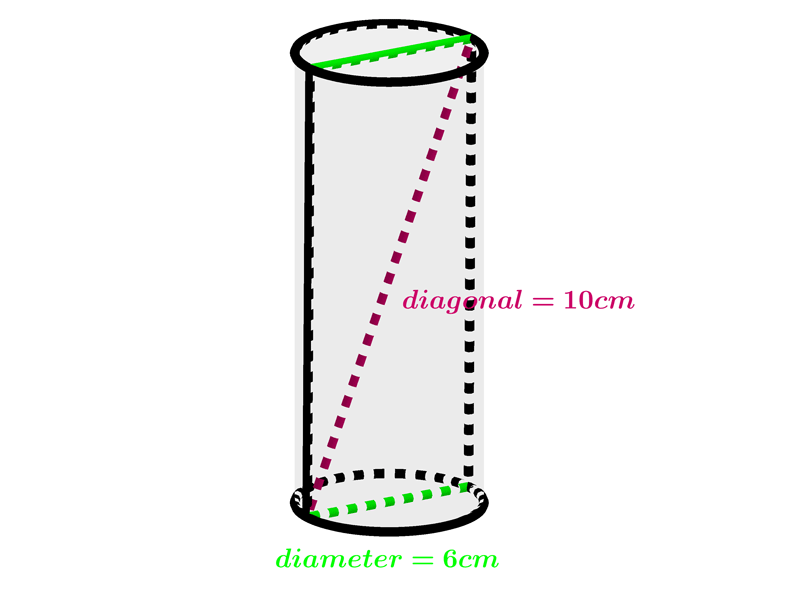$A=33 \pi cm ^{2}$ $A=66 \pi cm ^{2}$ $A=99 \pi cm ^{2}$
•  Question 13: 3 pts The following expression can be used to find the volume of the space between this two cylinders $$V=\left((27)^{2}-(20)^{2}\right)\pi \cdot 100 mm^{3}.$$